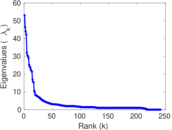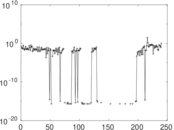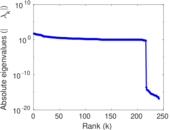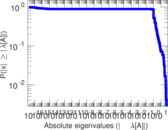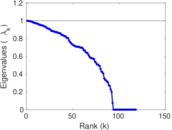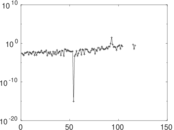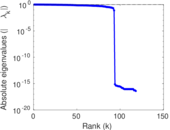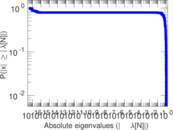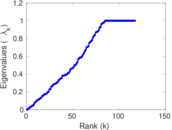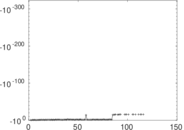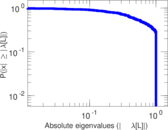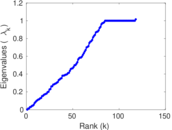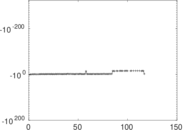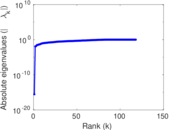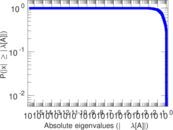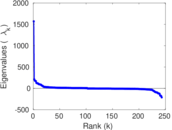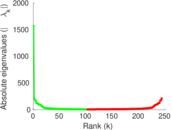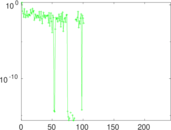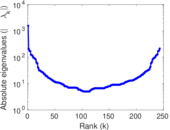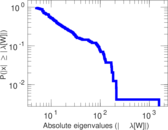# Wikibooks edits (ur)

This is the bipartite edit network of the Urdu Wikibooks. It contains users and pages from the Urdu Wikibooks, connected by edit events. Each edge represents an edit. The dataset includes the timestamp of each edit.

 Code `bur` Internal name `edit-urwikibooks` Name Wikibooks edits (ur) Data source http://dumps.wikimedia.org/ AvailabilityDataset is available for download Consistency checkDataset passed all tests Category Authorship network Dataset timestamp 2017-10-20 Node meaning User, article Edge meaning Edit Network formatBipartite, undirected Edge typeUnweighted, multiple edges Temporal dataEdges are annotated with timestamps

## Statistics

 Size n = 1,584 Left size n1 = 243 Right size n2 = 1,341 Volume m = 3,164 Unique edge count m̿ = 1,748 Wedge count s = 69,754 Claw count z = 3,441,284 Cross count x = 155,116,341 Square count q = 9,480 4-Tour count T4 = 358,464 Maximum degree dmax = 395 Maximum left degree d1max = 395 Maximum right degree d2max = 82 Average degree d = 3.994 95 Average left degree d1 = 13.020 6 Average right degree d2 = 2.359 43 Fill p = 0.005 364 22 Average edge multiplicity m̃ = 1.810 07 Size of LCC N = 1,205 Diameter δ = 13 50-Percentile effective diameter δ0.5 = 5.049 62 90-Percentile effective diameter δ0.9 = 7.535 41 Median distance δM = 6 Mean distance δm = 5.339 31 Gini coefficient G = 0.687 233 Balanced inequality ratio P = 0.234 987 Left balanced inequality ratio P1 = 0.139 697 Right balanced inequality ratio P2 = 0.317 320 Relative edge distribution entropy Her = 0.841 210 Power law exponent γ = 4.443 44 Tail power law exponent γt = 2.491 00 Tail power law exponent with p γ3 = 2.491 00 p-value p = 0.000 00 Left tail power law exponent with p γ3,1 = 1.841 00 Left p-value p1 = 0.270 000 Right tail power law exponent with p γ3,2 = 4.291 00 Right p-value p2 = 0.073 000 0 Degree assortativity ρ = −0.161 293 Degree assortativity p-value pρ = 1.175 78 × 10−11 Spectral norm α = 53.094 5 Algebraic connectivity a = 0.011 646 9 Spectral separation |λ1[A] / λ2[A]| = 1.145 06 Controllability C = 1,094 Relative controllability Cr = 0.716 907

## Plots

### Fruchterman–Reingold graph drawing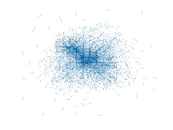### Degree distribution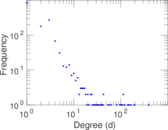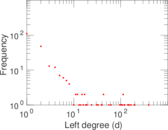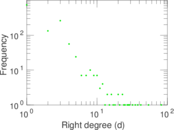### Cumulative degree distribution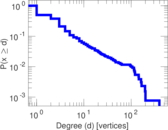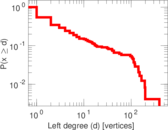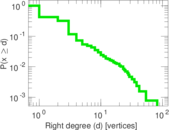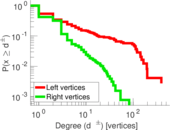### Lorenz curve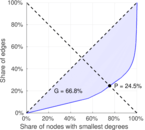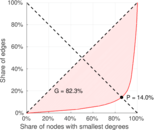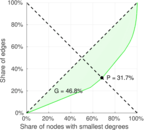### Spectral distribution of the adjacency matrix### Spectral distribution of the normalized adjacency matrix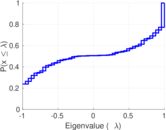### Spectral distribution of the Laplacian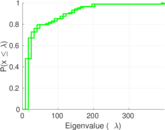### Spectral graph drawing based on the adjacency matrix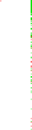### Spectral graph drawing based on the Laplacian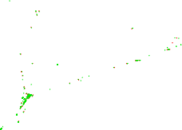### Spectral graph drawing based on the normalized adjacency matrix### Degree assortativity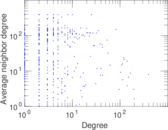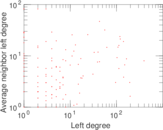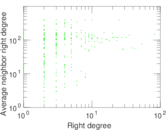### Zipf plot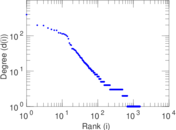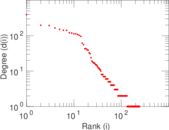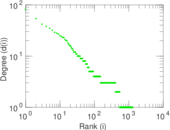### Hop distribution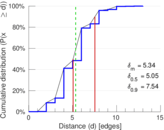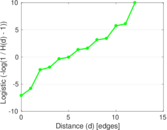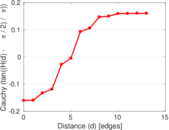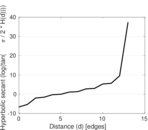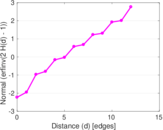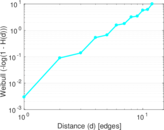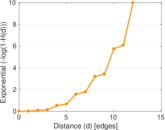### Double Laplacian graph drawing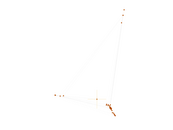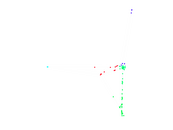### Delaunay graph drawing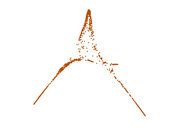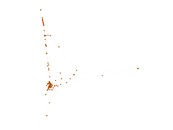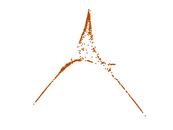### Edge weight/multiplicity distribution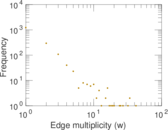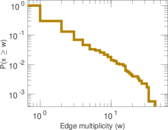### Temporal distribution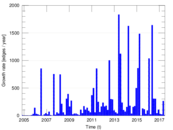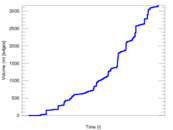### Temporal hop distribution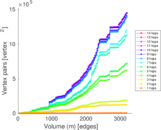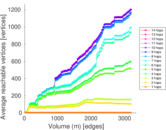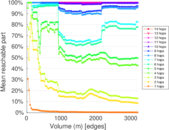### Diameter/density evolution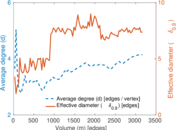### Matrix decompositions plots